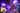## Neural Networks in JavaScript with deeplearn.js

A couple of my recent articles gave an introduction into a subfield of artificial intelligence by implementing foundational machine learning algorithms in JavaScript (e.g. linear regression with gradient descent , linear regression with normal equation or logistic regression with gradient descent ). These machine learning algorithms were…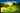## Logistic Regression with Gradient Descent in JavaScript

A couple of my recent articles gave an introduction to machine learning in JavaScript by solving regression problems with linear regression using gradient descent or normal equation . In a regression problem, an algorithm is trained to predict continuous values . It can be housing prices in a specific area based on a feature set such as…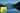## Multivariate Linear Regression, Gradient Descent in JavaScript

A recent article gave an introduction to the field of machine learning in JavaScript by predicting housing prices with gradient descent in a univariate regression problem. It used plain mathematical expressions and thus made use of the unvectorized implementation of gradient descent and the cost function. However, the unvectorized approach doesn…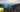## Gradient Descent with Vectorization in JavaScript

A recent article gave an introduction to the field of machine learning in JavaScript by predicting housing prices with gradient descent in a univariate regression problem. It used plain mathematical expressions and thus made use of the unvectorized implementation of gradient descent and the cost function. This article takes it one step further…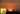## Improving Gradient Descent in JavaScript

A recent article gave an introduction to the field of machine learning in JavaScript by predicting housing prices with gradient descent in a univariate regression problem. There are several improvements to optimize the gradient descent algorithm that should be collected in this article. In general, when dealing with a regression problem, the…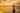## Host a single or multiple applications on Digital Ocean

There a various tutorials showing you how to host your web applications with a couple of lines on any hosting solution. But that's it. You are able to host your application somewhere, but there are a lot of open questions. What about using an own domain? What about securing it with SSL? What about hosting multiple applications side by side yet…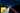## Polynomial Regression and Model Selection

After learning about gradient descent in a linear regression , I was curious about using different kinds of hypothesis functions to improve the result of the algorithm itself. So far, the hypothesis function was only a linear straight line. However, your training set may not have a linear correlation and by staying with a linear model it becomes…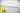## Linear Regression with Gradient Descent in JavaScript

Recently I started to take the Machine Learning course by Andrew Ng on Coursera. So far, it is a blast and I am so keen to apply all my learnings in math from university. Starting right into web development after university, I never had the opportunity to apply those learnings when implementing web applications. Now, it is refreshing to see use…## D3 on Angular: Small Multiples with Brushing

This article shows how you can achieve Small Multiples with D3 using Angular.js. According to Edward Tufte (1983) Small Multiples are a concept to visualize multiple homogenous visualizations like maps, line graphs or scatterplots. We will use this visualization concept to draw multiple line graphs with D3 on Angular. Here you can find an example…